# Test: Mensuration- 1

## 5 Questions MCQ Test IBPS PO Prelims- Study Material, Online Tests, Previous Year | Test: Mensuration- 1

Description
Attempt Test: Mensuration- 1 | 5 questions in 10 minutes | Mock test for Banking Exams preparation | Free important questions MCQ to study IBPS PO Prelims- Study Material, Online Tests, Previous Year for Banking Exams Exam | Download free PDF with solutions
QUESTION: 1

### Anil grows tomatoes in his backyard which is in the shape of a square. Each tomato takes 1 cm2 in his backyard. This year, he has been able to grow 131 more tomatoes than last year. The shape of the backyard remained a square. How many tomatoes did Anil produce this year?

Solution:

Let the area of backyard be x2 this year and y2 last year.

X2- Y2 = 131
(X+Y) (X-Y) = 131

Now, 131 is a prime number (a unique one too. Check out its properties on Google). Also, always identify the prime number given in a question. Might be helpful in cracking the solution.

⇒ (X+Y) (X-Y) = 131 x 1
⇒ X+Y = 131
⇒ X-Y = 1
⇒ 2X = 132
⇒ X = 66 and Y = 65

∴ Number of tomatoes produced this year = 662 = 4356

Choice (C) is therefore, the correct answer.

QUESTION: 2

### PQRS is a circle and circles are drawn with PO, QO, RO and SO as diameters. Areas A and B are marked. A/B is equal to: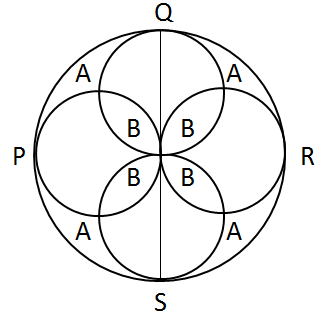Solution:Such questions are all about visualization and ability to write one area in terms of others.

Here, Let the radius of PQRS be 2r
∴ Radius of each of the smaller circles = 2r/2 = r

Area A can be written as:
A = π (2r)2 – 4 x π(r)2 (Area of the four smaller circles) + B (since, B has been counted twice in the previous subtraction)

A = 4πr2 - 4πr2 + B
A = B
A/B = 1

Choice (B) is therefore, the correct answer.

QUESTION: 3

### ABCD is a square drawn inside a square PQRS of sides 4 cm by joining midpoints of the sides PQ, QR, RS, SP. Another square is drawn inside ABCD similarly. This process is repeated infinite number of times. Find the sum of all the squares.

Solution: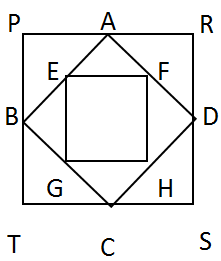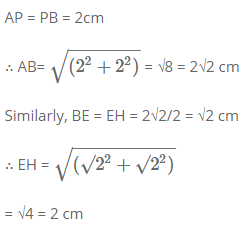If we write the infinite series of area of squares:
= 42 + (2√2)2 + 22 + ……. infinite

Since it is a decreasing series sum of infinite terms can be approximated.
= 16 + 8 + 4 +………infinite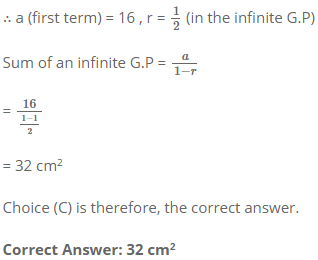QUESTION: 4

PQRST is a pentagon in which all the interior angles are unequal. A circle of radius ‘r’ is inscribed in each of the vertices. Find the area of portion of circles falling inside the pentagon.

Solution: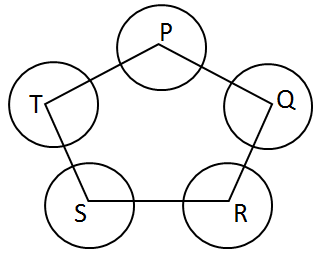Since neither angles nor sides are given in the question, immediately the sum of angles of pentagon should come in mind. To use it,

We know the area of the sectors of a circle is given as: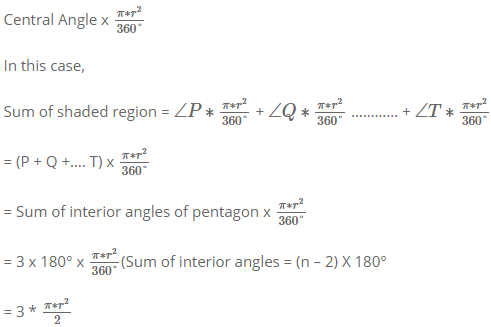Note: The above concept is applicable for a polygon of n sides.

Choice (B) is therefore, the correct answer.

QUESTION: 5

Raj stands in a corner of his square farm. Angle of elevation of a scarecrow placed in diagonally opposite corner is 60°. He starts walking backwards in a straight line and after 80ft he realizes that angle of elevation of the scarecrow now is 30°. What is area of the field?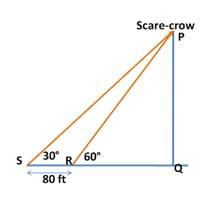Solution: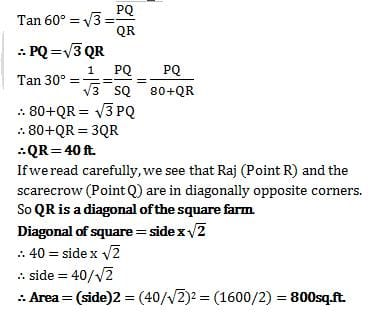Use Code STAYHOME200 and get INR 200 additional OFF Use Coupon Code